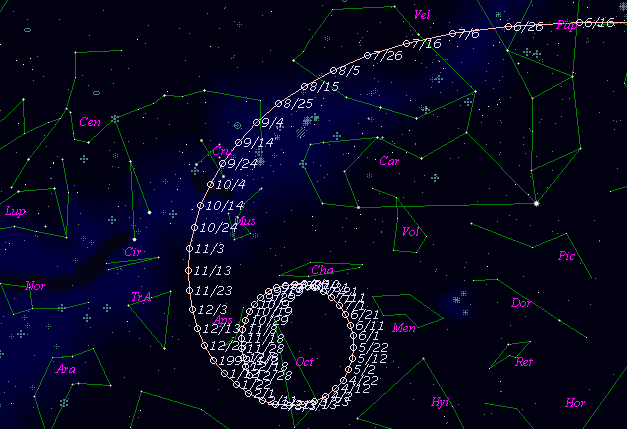# \$B%=!<%[!

C/1998 J1 ( SOHO )###\$B%W%m%U%#!<%k(B

 \$BH/8+F|(B 1998\$BG/(B5\$B7n(B3\$BF|(B \$BH/8+8wEY(B 0\$BEy0J>e(B \$BH/8+ SOHO-LASCO coronagraph C3

###\$B###\$B50F;MWAG(B

```   The following improved orbital elements by Kenji Muraoka, are
from 196 observations  1998 May 20  to  Aug.  5, perturbations
by 9 Planets, Moon and 5 minor planets were taken into account.
The mean residual is +/- 0.70 arc seconds.

Epoch  =  1998 May  27.0  TT       JDT = 2450960.5
T  =  1998 May   8.61890       +/- 0.00016 (m.e.) TT
Peri. =  110.55820                +/- 0.00095
Node  =  351.64727                +/- 0.00173   (2000.0)
Incl. =   62.93174                +/- 0.00019
q  =    0.1532152              +/- 0.0000005 AU
e  =    1.0001781              +/- 0.0000134
1/a  =   -0.0011623              +/- 0.0000875 1/AU
orig. 1/a  =   -0.000434  1/AU
fut.  1/a  =   -0.001014  1/AU
```

###\$B@1?^(B###\$B8wEYJQ2=(B

```        m1 = 5.6 + 5 log\$B&\$(B + 10.0 log r  [  , 0]  (              \$B!A(B1998\$BG/(B 5\$B7n(B 8\$BF|(B)
m1 = 6.2 + 5 log\$B&\$(B +  6.0 log r  [ 0,50]  (1998\$BG/(B 5\$B7n(B 8\$BF|!A(B1998\$BG/(B 6\$B7n(B27\$BF|(B)
m1 = 4.0 + 5 log\$B&\$(B + 20.0 log r  [50,  ]  (1998\$BG/(B 6\$B7n(B27\$BF|!A(B              )
```##### \$B50F;MWAG\$OB<2,7r<#;a\$N7W;;\$K\$h\$k\$b\$N\$G\$9!#(B \$B@1?^\$O(B StellaNavigator Ver.2.0 for Windows (\$B%"%9%H%m%"!<%D(B \$BJTCx(B / \$B%"%9%-!<=PHG6I4)(B) \$B\$G:n@.\$7\$?\$b\$N\$G\$9!#(B \$B8wEY%0%i%U\$O(BComet for Windows\$B\$G:n@.\$7\$?\$b\$N\$G\$9!#(B